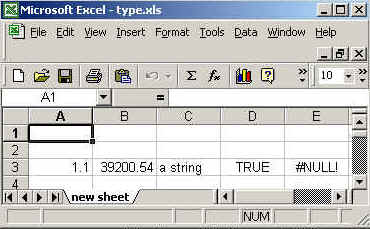Tutorials

# Passing Various Data Types into Cells

In this program, we are going to pass various data types into the cells and rows in the excel sheet using java .

In this program, we are going to pass various data types into the cells and rows in the excel sheet using java .

# passing various data types into cells

In this program, we are going to pass various data types into the cells and rows in the excel sheet using java .You can create any number of cells and rows and also you can pass different data type values into cells.

The package we need to import is  :
java.io.*,
org.apache.poi.hssf.usermodel.HSSFSheet,
org.apache.poi.hssf.usermodel.HSSFCell,
org.apache.poi.hssf.usermodel.HSSFRow,
org.apache.poi.hssf.usermodel. HSSFWorkbook

org.apache.poi.hssf.usermodel.HSSFRow class is used to create  rows  in which we can set the cells and add the values.

org.apache.poi.hssf.usermodel.HSSFCell class is used to create a new cell and add the values into cells.

createRow((short)value):
This method is used to create  a new row. The argument "value" (short type) is the number for which we are going to create
the row e.g. createRow((short)2) is for creating 2th row.

createCell((short)value):
This method is used to create a new cell. The argument "value" (short type) is the number for which we are going to create the new cell  e.g. createCell((short)0) is for creating 0th cell.

setCellValue(values):
This method is used to add the value into the cell. You can add any data types into cell by using this method. In this example, we have used four data types int , float , string and boolean which are being added at 0th ,1st,2nd,3th   and 4th cells  into 2th row.

In this example, at 0th cell we are passing float values ,at 1st cell we are passing date, at 2nd cell we are passing string ,at 3rd cell we are passing boolean value and at 4th position we are passing HSSFCell.CELL_TYPE_ERROR it will be null value as no error will occur.

The code of the program is given below:

 ```<%@ page import="org.apache.poi.hssf.usermodel.HSSFSheet"%> <%@ page import="org.apache.poi.hssf.usermodel.HSSFWorkbook"%> <%@ page import="org.apache.poi.hssf.usermodel.HSSFRow"%> <%@ page import="org.apache.poi.hssf.usermodel.HSSFCell"%> <%@ page import="java.io.*" %> <%@ page import="java.util.*" %> <% try{  HSSFWorkbook hwb = new HSSFWorkbook();  HSSFSheet sheet = hwb.createSheet("new sheet");  HSSFRow row = sheet.createRow((short)2);  row.createCell((short) 0).setCellValue(1.1);  row.createCell((short) 1).setCellValue(new Date());  row.createCell((short) 2).setCellValue("a string");  row.createCell((short) 3).setCellValue(true);  row.createCell((short) 4).setCellType(HSSFCell.CELL_TYPE_ERROR);  FileOutputStream fileOut = new FileOutputStream ("c:\\excel\\type.xls");  hwb.write(fileOut);  fileOut.close();  out.println("Your excel file has been generated");      } catch ( Exception ex ) {      }  %>```

The output of the program is given below: